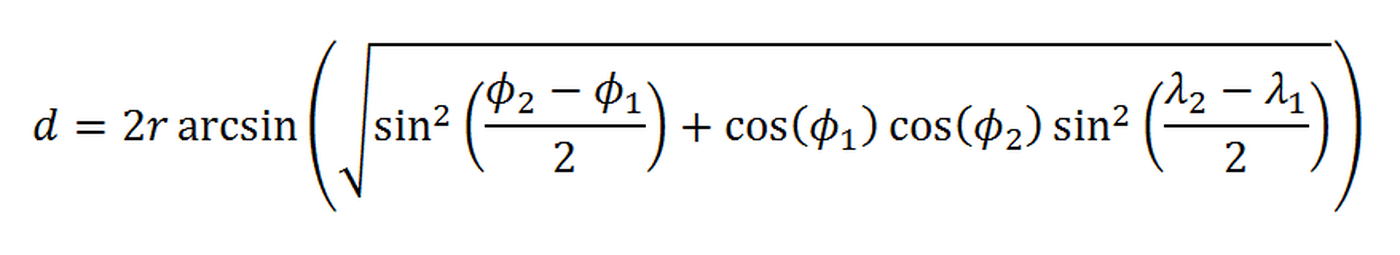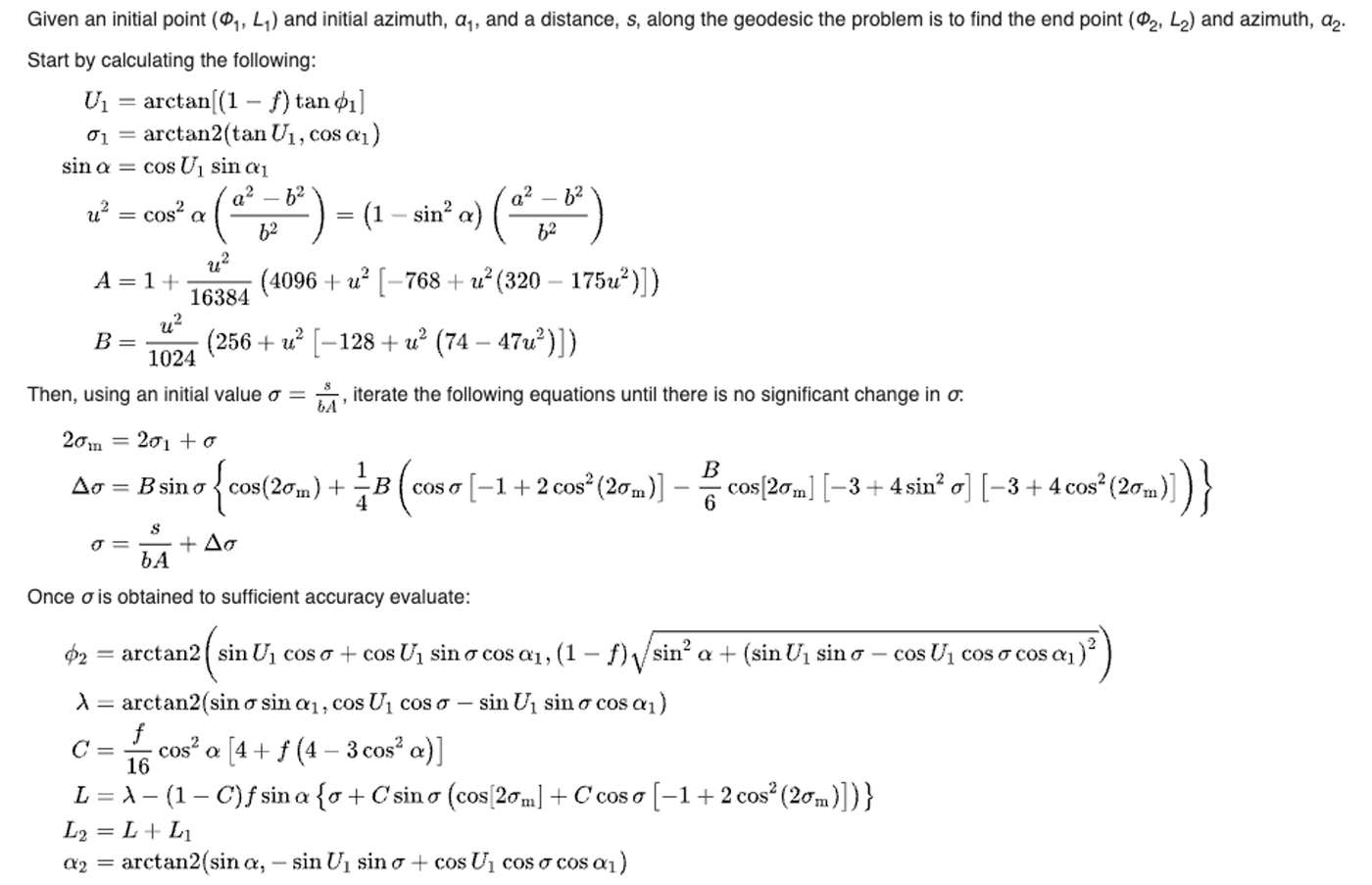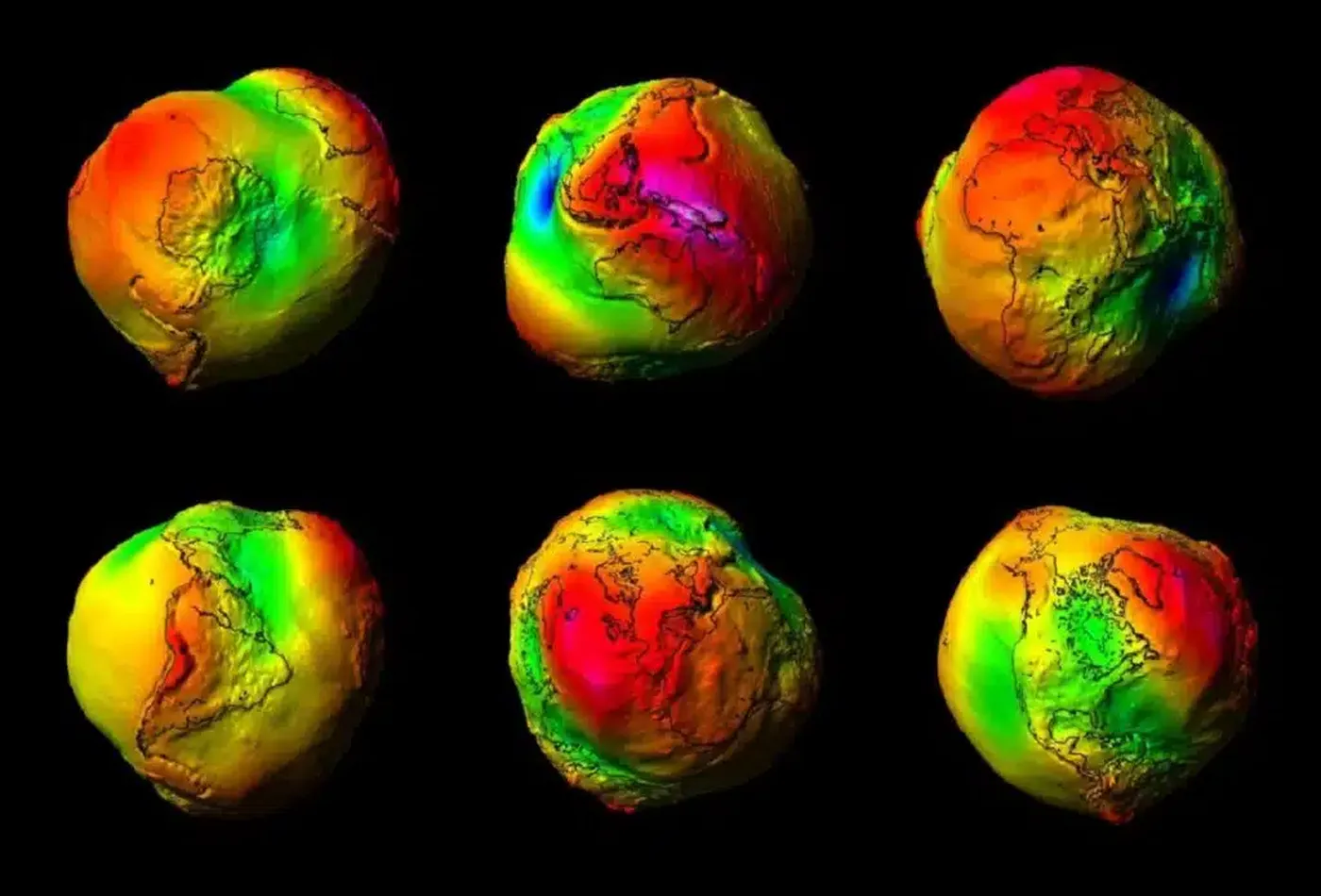Algorithms
October 28, 2023

# How Geosearch WorksWith Orama v2.0.0-beta.1, we released the first, experimental API for allowing Geosearch queries.

Our demo made a great success, and we’d like to take some time to explain how we’re able to run geosearch queries so fast.

Remember that Orama is open source, so you can inspect the code as you read this post! Don’t forget to star the repository, it helps us a lot.

## What is a Geosearch query?

Geosearch queries are a specialized type of search that enables users to discover geographical locations using specific coordinates. This powerful tool is often integrated into applications that rely on location-based data. For example, mapping or navigation software heavily relies on Geosearch queries to provide precise location details. By inputting a set of coordinates, the system can pinpoint the exact location on a map. This functionality is also available on real estate platforms, delivery tracking systems, ride-sharing apps, and some social media platforms that offer location tagging.

Moreover, Geosearch queries are not just about finding a specific location. They can also identify places within a certain radius of a given point, which has significant applications in location-based marketing, environmental studies, and urban planning, among others.

We have incorporated this feature into Orama `v2.0.0-beta.1`, allowing developers to harness the power of Geosearch in their own applications.

## How does it work?Here is where things get a bit more technical. Orama uses a highly optimized data structure called BKD Tree to index and retrieve geopoints on a 2D surface (in our case, the earth’s surface).

We have already published a long, detailed blog post on how BKD Trees works, and we highly recommend you read it if you’re interested in algorithms and data structures!

In Orama, geosearch works in two different modes:

1. Distance-based search
2. Bounding-box-based search

These two functionalities bring different complexities, and we will now try to analyze them.

``````1export function searchByRadius (
2  node: Nullable<Node>,
3  center: Point,
5  inclusive = true,
6  sort: SortGeoPoints = 'asc',
7  highPrecision = false
8): GeoSearchResult[] {
9  const distanceFn = highPrecision ? vincentyDistance : haversineDistance
10  const stack: Array<{ node: Nullable<Node>, depth: number }> = [{ node, depth: 0 }]
11  const result: GeoSearchResult[] = []
12
13  while (stack.length > 0) {
14    const { node, depth } = stack.pop() as { node: Node, depth: number }
15    if (node === null) continue
16    const dist = distanceFn(center, node.point)
17
18    if (inclusive ? dist <= radius : dist > radius) {
19      result.push({ point: node.point, docIDs: node.docIDs ?? [] })
20    }
21
22    if (node.left != null) {
23      stack.push({ node: node.left, depth: depth + 1 })
24    }
25    if (node.right != null) {
26      stack.push({ node: node.right, depth: depth + 1 })
27    }
28  }
29
30  if (sort) {
31
32    result.sort((a, b) => {
33      const distA = distanceFn(center, a.point)
34      const distB = distanceFn(center, b.point)
35      return sort.toLowerCase() === 'asc' ? distA - distB : distB - distA
36    })
37  }
38
39  return result
40}
41``````

The goal of radius-based search is to find all points within a given radius from the center geopoint (specified by longitude and latitude) in meters.

As we mentioned in the BKD Trees Explained blog post, we don’t want to use recursion, which slows down computations and can cause stack overflows on big datasets.

This makes things a bit more difficult, but the performance rewards make it definitely worth it.

The traversal process starts with a stack and a result list. The stack holds nodes for inspection, and the result list holds points that meet our search criteria. The function starts by adding the initial node to the stack with a depth of `0`.

The function then uses a depth-first traversal to explore the BKD Tree. This continues until all nodes have been inspected. Each node's distance to the specified center is calculated using a chosen distance formula, which we will discuss later. This formula will reliably provide the shortest distance between two points on a sphere's surface.

The function then compares this distance to our defined radius. If the distance falls within the radius (also determined by the `inclusive` parameter), the point from the node is added to the result list.

If a node has left or right children, these are added to the stack with an incremented depth value. This ensures all potential points are evaluated.

Once finished, the function returns the list of points. Each point in this list shows the function's ability to identify locations in the BKD Tree that are within the given radius from the center.

## Search By Polygon

``````1export function searchByPolygon (root: Nullable<Node>, polygon: Point[], inclusive = true, sort: SortGeoPoints = null, highPrecision = false): GeoSearchResult[] {
2  const stack: SearchTask[] = [{ node: root, depth: 0 }]
3  const result: GeoSearchResult[] = []
4
5  while (stack.length > 0) {
8
9    const { node, depth } = task
10    const nextDepth = depth + 1
11
12    if (node.left != null) {
13      stack.push({ node: node.left, depth: nextDepth })
14    }
15
16    if (node.right != null) {
17      stack.push({ node: node.right, depth: nextDepth })
18    }
19
20    const isInsidePolygon = isPointInPolygon(polygon, node.point)
21
22    if (isInsidePolygon && inclusive) {
23      result.push({ point: node.point, docIDs: node.docIDs ?? [] })
24    } else if (!isInsidePolygon && !inclusive) {
25      result.push({ point: node.point, docIDs: node.docIDs ?? [] })
26    }
27  }
28
29  const centroid = calculatePolygonCentroid(polygon)
30
31  if (sort) {
32    const sortFn = highPrecision ? vincentyDistance : haversineDistance
33
34    result.sort((a, b) => {
35      const distA = sortFn(centroid, a.point)
36      const distB = sortFn(centroid, b.point)
37      return sort.toLowerCase() === 'asc' ? distA - distB : distB - distA
38    })
39  }
40
41  return result
42}``````

Searching by polygon-based boundary enables users to locate specific points within a defined polygonal boundary using a BKD Tree.

At the start of the function, two lists are created: a stack and a results list. The stack holds nodes that need to be examined while the results list collects the points that fall within the defined polygon. The process begins with the root node of the BKD Tree, which is added to the stack.

To navigate the BKD Tree, we use a depth-first traversal, ensuring each node is evaluated. When a node is encountered, the function checks if the point it contains is within the given polygon. This is done using a reliable algorithm that can determine if a point is within a polygon.

If a node's point is found to be within the polygon, it's added to the results list. However, the function still needs to check the node's children. Just because a node is within the polygon doesn't mean its children will be, and vice versa. So, the function adds both the left and right children of the node to the stack—if they exist.

Once all nodes have been evaluated and the stack is empty, the function has one last task. If the user wants the results ordered by a specific attribute, the function will sort the results list as requested.

## If only the earth was flat…

A common issue while calculating the distance between two points on a planet is their non-spherical shape.

Planets, in fact, are ellipsoids, slightly flattened at the poles and stretched at the equator. This leads to variations in distance between two points based on their latitudes.

To address this, we employ two different distance calculation methods: the Haversine Distance and Vincenty's Formulae.

Haversine Distance is simpler and faster but less accurate, especially for long distances. Conversely, Vincenty's Formulae is more complex and slower but provides a higher level of accuracy by considering the Earth's shape. Users can select the method that best suits their requirements by setting the `highPrecision` property to `true` when using geosearch (official documentation here).

How do these two formulas differ? Let’s find out.

### The Haversine DistanceDerived from the trigonometric haversine function or "half-versed sine," the formula first converts the latitude and longitude of both points from degrees to radians. This conversion is crucial because trigonometric functions in most programming languages expect input in radians.

After determining the difference in latitudes and longitudes, the Haversine formula employs these values to compute the shortest distance over the Earth's surface between the two points, resulting in a value often used in navigation and geospatial analysis.

As previously mentioned, the Haversine formula is simple and fast. However, it is not very accurate when calculating the distance between two points on irregularly shaped spheres, such as the Earth.

### Vincenty FormulaeThe Vincenty formulae offer a method to calculate the distance between two points on the surface of an ellipsoid, making them especially suitable for precise geodetic computations on Earth, which isn't a perfect sphere. Developed by Thaddeus Vincenty in 1975, these formulae use iterative methods to determine the ellipsoidal distance.

The process starts by representing the Earth as an oblate spheroid, which acknowledges the Earth's flattened poles and bulging equator. Given the latitude and longitude of two points, the Vincenty formulae compute the shortest path, or geodesic, between them on the ellipsoid's surface.

This method is more accurate than the haversine formula for computing distances on Earth due to its consideration of the planet's elliptical shape. However, its iterative nature means it can be slower and, in rare cases, fail to converge to a solution.

### But the Earth is not an ellipsoid either!That is very true, the Earth’s shape is technically a geoid.

A geoid is a gravitational equipotential surface that best represents the mean sea level. This surface is irregular and does not have a mathematical formula that perfectly describes it. However, for most practical purposes involving short distances, treating the Earth as an ellipsoid or even a sphere provides a sufficiently accurate approximation.

For larger distances or more precise calculations, more complex models and calculations are necessary, considering factors such as variations in the Earth's gravitational field.

## High-precision Geosearch in Orama

Although Earth is not a perfect sphere or an ellipsoid, most tests show that the Haversine distance is sufficient for the majority of Geosearch queries.

For those who need to calculate very long distances with greater precision, Orama `v2.0.0-beta.2` introduces the `highPrecision` parameter for Geosearch. This allows you to switch the distance function from Haversine to Vincenty.

The API is very intuitive and works as follows:

``````1import { create, insert, search } from '@orama/orama'
2
3const db = await create({
4  schema: {
5    id: 'string',
6    location: 'geopoint',
7	} as const
8})
9
10await insert(db, { id: '1', location: { lat: -50.6964111, lon: 70.2120854 } })
11await insert(db, { id: '2', location: { lat: -50.7403564, lon: 70.1823094 } })
12await insert(db, { id: '3', location: { lat: -51.2512207, lon: 70.1123535 } })
13await insert(db, { id: '4', location: { lat: -50.8639526, lon: 70.0796264 } })
14await insert(db, { id: '5', location: { lat: -50.6167603, lon: 70.0973989 } })
15
16const polygonResults = await search(db, {
17  where: {
18    location: {
19      polygon: {
20	      coordinates: [
21          { lat: -52.6779842, lon: 71.5489379 },
22	        { lat: -52.9086971, lon: 71.2828433 },
23          { lat: -51.8759823, lon: 71.2086670 },
24          { lat: -51.5024471, lon: 71.4932231 },
25	        { lat: -52.6779842, lon: 71.5489379 },
26	      ],
27        inside: false,
28        highPrecision: true // Uses the Vincenty Formulae instead of Haversine
29      }
30    }
31  }
32})``````

## Conclusion

Orama `v2.0.0-beta.1` introduces a Geosearch feature, enabling users to discover geographical locations using specific coordinates. Geosearch queries can identify places within a certain radius of a given point or within a defined polygonal boundary. The system uses a highly optimized data structure called BKD Tree to index and retrieve geopoints. Two different distance calculation methods, Haversine Distance and Vincenty's Formulae, are employed to account for the Earth's non-spherical shape. The `highPrecision` parameter allows users to switch between these methods based on their precision needs.

If you enjoyed this blog post, try the Orama Geosearch live demo, and remember to star our repository, it means a lot to us!

### Posted byMichele Riva

CTO @OramaSearch